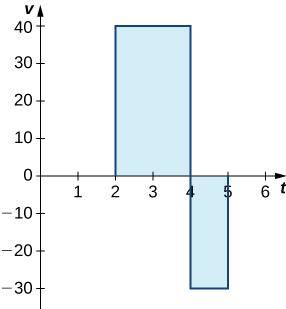## Net Change Theorem

### Learning Outcomes

• Apply the basic integration formulas
• Explain the significance of the net change theorem
• Use the net change theorem to solve applied problems

## Basic Integration Formulas

Recall the integration formulas given in the Table of Antiderivatives and the rule on properties of definite integrals. Let’s look at a few examples of how to apply these rules.

### Example: Integrating a Function Using the Power Rule

Use the power rule to integrate the function ${\displaystyle\int }_{1}^{4}\sqrt{t}(1+t)dt.$

Watch the following video to see the worked solution to Example: Integrating a Function Using the Power Rule.

### Try It

Find the definite integral of $f(x)={x}^{2}-3x$ over the interval $\left[1,3\right].$

## The Net Change Theorem

The net change theorem considers the integral of a rate of change. It says that when a quantity changes, the new value equals the initial value plus the integral of the rate of change of that quantity. The formula can be expressed in two ways. The second is more familiar; it is simply the definite integral.

### Net Change Theorem

The new value of a changing quantity equals the initial value plus the integral of the rate of change:

$\begin{array}{}\\ \\ F(b)=F(a)+{\displaystyle\int }_{a}^{b}F\text{‘}(x)dx\hfill \\ \hfill \text{or}\hfill \\ {\displaystyle\int }_{a}^{b}F\text{‘}(x)dx=F(b)-F(a).\hfill \end{array}$

Subtracting $F(a)$ from both sides of the first equation yields the second equation. Since they are equivalent formulas, which one we use depends on the application.

The significance of the net change theorem lies in the results. Net change can be applied to area, distance, and volume, to name only a few applications. Net change accounts for negative quantities automatically without having to write more than one integral. To illustrate, let’s apply the net change theorem to a velocity function in which the result is displacement.

We looked at a simple example of this in The Definite Integral. Suppose a car is moving due north (the positive direction) at 40 mph between 2 p.m. and 4 p.m., then the car moves south at 30 mph between 4 p.m. and 5 p.m. We can graph this motion as shown in (Figure).Figure 1. The graph shows speed versus time for the given motion of a car.

Just as we did before, we can use definite integrals to calculate the net displacement as well as the total distance traveled. The net displacement is given by

$\begin{array}{cc}{\displaystyle\int }_{2}^{5}v(t)dt\hfill & ={\int }_{2}^{4}40dt+{\displaystyle\int }_{4}^{5}-30dt\hfill \\ & =80-30\hfill \\ & =50.\hfill \end{array}$

Thus, at 5 p.m. the car is 50 mi north of its starting position. The total distance traveled is given by

$\begin{array}{}\\ \\ {\displaystyle\int }_{2}^{5}|v(t)|dt\hfill & ={\int }_{2}^{4}40dt+{\displaystyle\int }_{4}^{5}30dt\hfill \\ & =80+30\hfill \\ & =110.\hfill \end{array}$

Therefore, between 2 p.m. and 5 p.m., the car traveled a total of 110 mi.

To summarize, net displacement may include both positive and negative values. In other words, the velocity function accounts for both forward distance and backward distance. To find net displacement, integrate the velocity function over the interval. Total distance traveled, on the other hand, is always positive. To find the total distance traveled by an object, regardless of direction, we need to integrate the absolute value of the velocity function.

### Example: Finding Net Displacement

Given a velocity function $v(t)=3t-5$ (in meters per second) for a particle in motion from time $t=0$ to time $t=3,$ find the net displacement of the particle.

### Example: Finding the Total Distance Traveled

Use (Figure) to find the total distance traveled by a particle according to the velocity function $v(t)=3t-5$ m/sec over a time interval $\left[0,3\right].$

Watch the following video to see the worked solution to Example: Finding the Total Distance Traveled.

### Try It

Find the net displacement and total distance traveled in meters given the velocity function $f(t)=\frac{1}{2}{e}^{t}-2$ over the interval $\left[0,2\right].$

## Applying the Net Change Theorem

The net change theorem can be applied to the flow and consumption of fluids, as shown in the example below.

### Example: How Many Gallons of Gasoline Are Consumed?

If the motor on a motorboat is started at $t=0$ and the boat consumes gasoline at a rate which can be modeled for the first two hours as $5-\frac{t^{3}}{100}$ gal/hr for the first hour, how much gasoline is used in the first hour?

### Try It

Suppose that, instead of remaining steady during the second half hour of Andrew’s outing, the wind starts to die down according to the function $v(t)=-10t+15.$ In other words, the wind speed is given by

$v(t)=\bigg\{\begin{array}{lll}20t+5\hfill & \text{ for }\hfill & 0\le t\le \frac{1}{2}\hfill \\ -10t+15\hfill & \text{ for }\hfill & \frac{1}{2}\le t\le 1.\hfill \end{array}$

Under these conditions, how far from his starting point is Andrew after 1 hour?